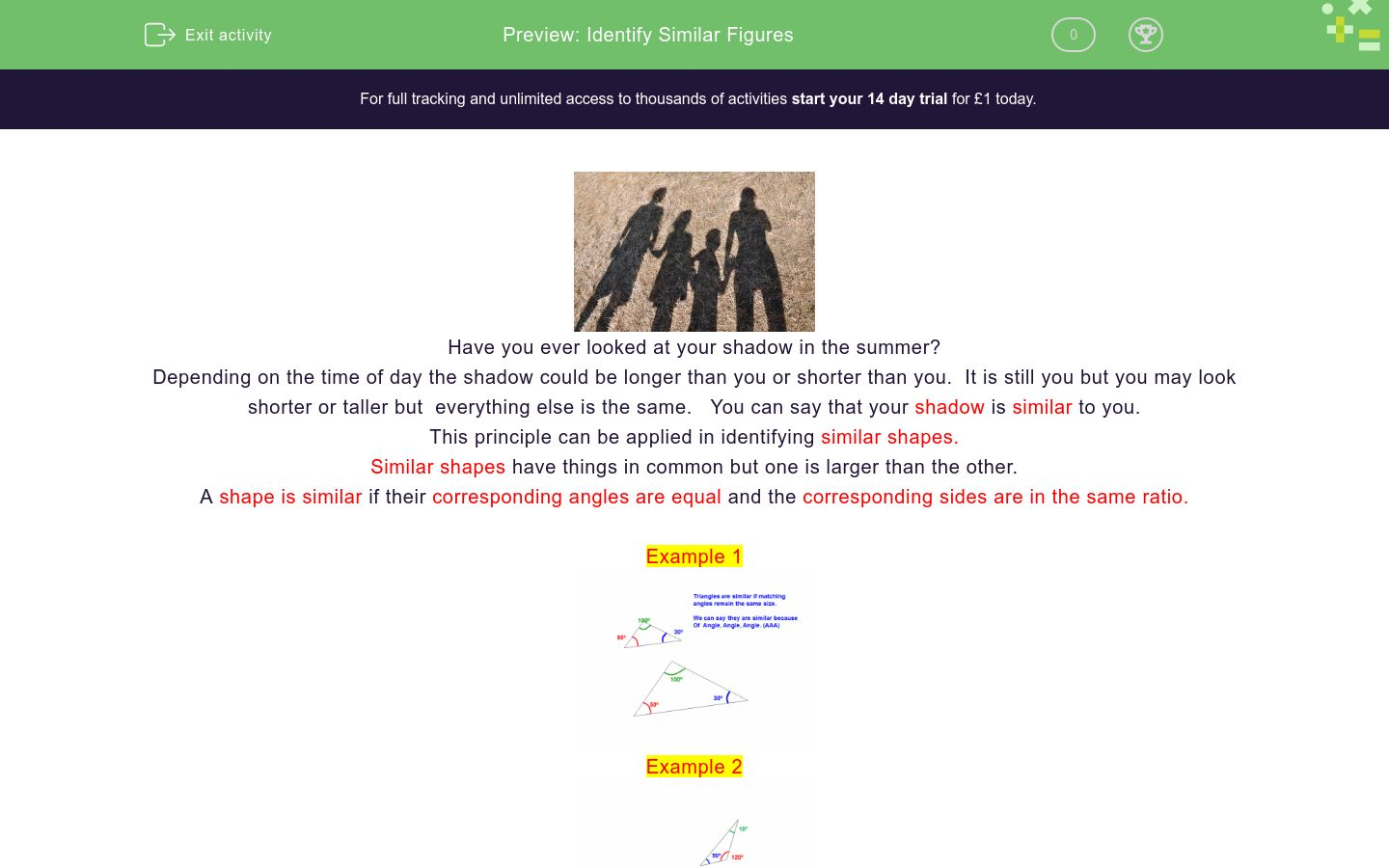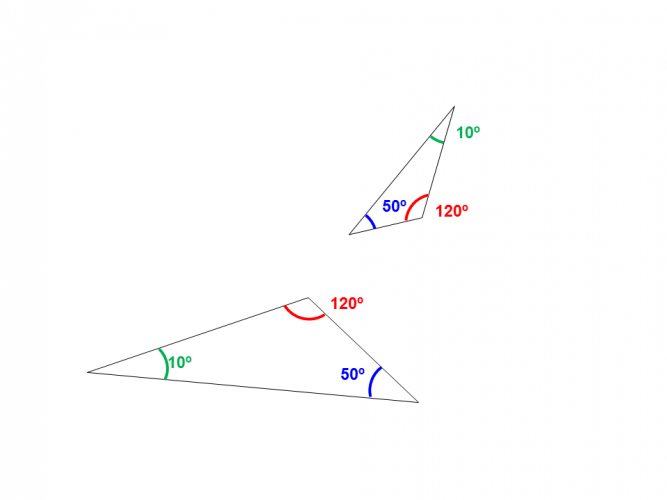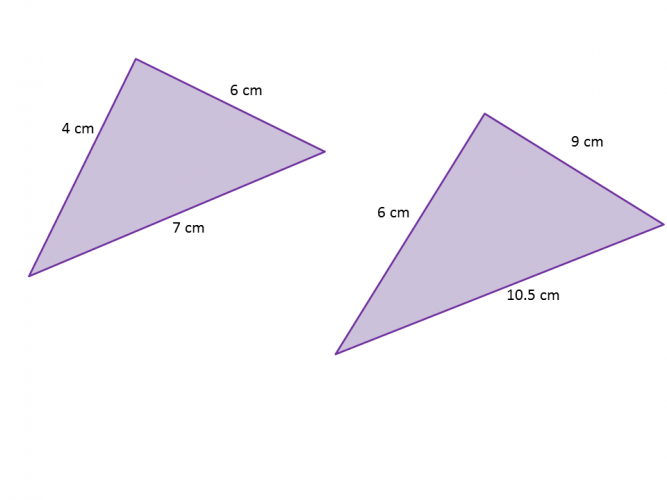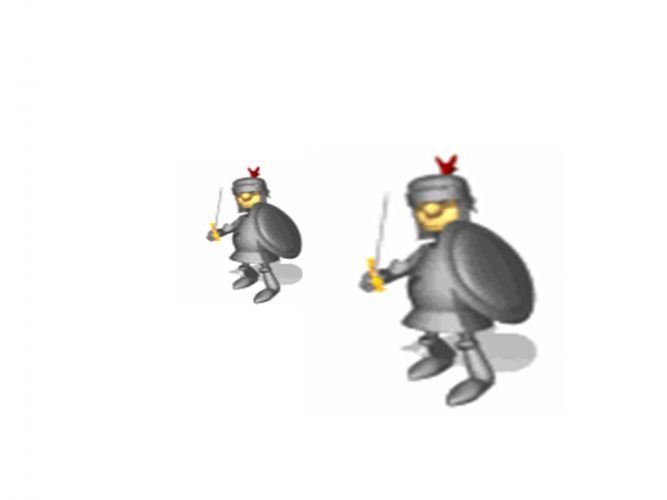# Identify Similar Figures

In this worksheet, students will identify similar shapes.Key stage:  KS 4

GCSE Subjects:   Maths

GCSE Boards:   AQA, Eduqas, Pearson Edexcel, OCR

Curriculum topic:   Geometry and Measures, Congruence and Similarity

Curriculum subtopic:   Mensuration and Calculation, Similarity

Difficulty level:### QUESTION 1 of 10Depending on the time of day the shadow could be longer than you or shorter than you.  It is still you but you may look shorter or taller but  everything else is the same.   You can say that your shadow is similar to you.

This principle can be applied in identifying similar shapes.

Similar shapes have things in common but one is larger than the other.

A shape is similar if their corresponding angles are equal and the corresponding sides are in the same ratio.

Example 1Example 2Make sure that when triangles are rotated the angles still correspond.

You only need to be given two corresponding angles to decide if a shape is similar.

If two corresponding angles are the same then, the third angle must be the same because of the rule that angles in a triangle add up to 180°.

Example 3Example 4And now over to youA and B

B and C

A and CWhich shape is the odd one out?Which one of the following is true?

Similar SAS

Similar AAA

Similar SSS

Not similarSimilar SAS

Similar AAA

Similar SSS

Not similarThe smaller playing card is 133 mm by 72 mm and the larger playing card is 165 mm by 82 mm

 Similar Not similar Playing cardsWhich way can you check to see if a shape is similar?

Side, Angle, Side, (SAS)

Side, Side, Side, (SSS)

Angle, Side, Angle (ASA)

Angle, Angle, Angle (AAA)These triangles are similar, not similar.True False These quadrilaterals are similarComplete the following sentence

 True False These quadrilaterals are similarWhich of the following must be similar to each other?

a) Two equilateral triangles

b) Two rectangles

c) Two isosceles triangles

d) Two squares

e) Two regular pentagons

f) Two Kites

g) Two rhombuses

• Question 1A and C
EDDIE SAYS
To find out if these are similar you will need to look at the ratio of the corresponding sides. 18 ÷ 6 = 3 18 ÷ 6 = 3 15 ÷ 5 = 3 Did you know that Lord Nelson was 5 ft 6 inches and his statue is 17 ft 4 inches. Thats Horatio of 3: 1.
• Question 2Which shape is the odd one out?

D
EDDIE SAYS
The angle 67° is not within any of the other triangles. If you are unsure which one, work out one of the missing angles to see if you can spot which triangle it may be. If in doubt always use what you know to try and help.
• Question 3Which one of the following is true?

Similar SSS
EDDIE SAYS
Make sure you take the time to calculate all corresponding sides to be extra sure. You don't want to work out 2 and assume the third side is the same ratio. That wouldn't be good would it.
• Question 4EDDIE SAYS
If you know the triangles are similar because you are told in the question, then all you need to do is divide one larger corresponding side by the smaller one. Always great when you only have to do one calculation.
• Question 5The smaller playing card is 133 mm by 72 mm and the larger playing card is 165 mm by 82 mm

 Similar Not similar Playing cards
EDDIE SAYS
By dividing the larger sides by the smaller sides you can see that the ratios come out differently, so therefore not similar. 165 ÷ 133 = 1.24 mm 82 ÷ 72 = 1.14 mm
• Question 6Which way can you check to see if a shape is similar?

Side, Angle, Side, (SAS)
Side, Side, Side, (SSS)
Angle, Side, Angle (ASA)
Angle, Angle, Angle (AAA)
EDDIE SAYS
Yes of course you can use them all. Don't you just love it when you have options? The only thing to be careful of here is to make sure the angles and sides you are looking at are corresponding to each other.
• Question 7These triangles are similar, not similar.
EDDIE SAYS
Be careful here. Although you have been given two angles that are the same, they are not corresponding to each other.
• Question 8True False These quadrilaterals are similar
EDDIE SAYS
Although the 90° and the 140° are corresponding to each other, the 60° and 70° are not.
• Question 9Complete the following sentence

EDDIE SAYS
How did you decide on this one? The 80° and the 100° are clearly corresponding. What we have to decide is whether the other two angles are correct? Use your knowledge about what you know about angles in a quadrilateral. i.e they add up to 360°. Take any shape and find the missing angle. If the missing angle is in the other shape then of course it is corresponding. 100 + 50 + 80 = 230° 360 - 230 = 130° which corresponds with the other shape.
• Question 10Which of the following must be similar to each other?

a) Two equilateral triangles

b) Two rectangles

c) Two isosceles triangles

d) Two squares

e) Two regular pentagons

f) Two Kites

g) Two rhombuses

a, d, e
EDDIE SAYS
This draws on your knowledge of shapes. Equilateral means the angles and sides are the same, so they will always be in the same ratio. This is the same for regular shapes. Squares sides are always the same and the angles are all 90° so this has to be similar. The other shapes angles may correspond but sides may have a different ratio. (although they could be similar but you would have to look at the angles and work out the ratio to check).
---- OR ----

Sign up for a £1 trial so you can track and measure your child's progress on this activity.

### What is EdPlace?

We're your National Curriculum aligned online education content provider helping each child succeed in English, maths and science from year 1 to GCSE. With an EdPlace account you’ll be able to track and measure progress, helping each child achieve their best. We build confidence and attainment by personalising each child’s learning at a level that suits them.

Get started